Function Repository Resource:

# CausalConnectionGraph

Generate a graph giving the relations between regions of causally equivalent events in a causal graph

Contributed by: Stephen Wolfram with Jonathan Gorard and Max Piskunov
 ResourceFunction["CausalConnectionGraph"][cgf,ti,tf] generates a graph of relations between causally equivalent regions that are detected at step ti in the causal graph generated by cgf, as determined by outcomes at step tf. ResourceFunction["CausalConnectionGraph"][cgf,{ti1,ti2},{tf1,tf2}] considers regions detected between steps ti1 and ti2, with outcomes determined by looking at steps tf1 through tf2. ResourceFunction["CausalConnectionGraph"][cg,{vi1,…},{vf1,…}] generates the causal connection graph from the causal graph cg by considering causally equivalent regions among the event nodes vik, as determined by looking at the event nodes vfk. ResourceFunction["CausalConnectionGraph"][c,i,f,"Full"] gives the full causal connection graph for every event, not aggregating causally equivalent events.

## Details and Options

If the future light cone of A contains the future light cone of B, then there is a directed edge from A to B in the resulting graph.
If the future light cone of A intersects the future light cone of B, but neither contains the other, then there is an undirected edge between A and B in the resulting graph.
Typical forms for the causal graph generator include:
 ResourceFunction[“SubstitutionSystemCausalGraph”][rules,init, #] & string substitution system ResourceFunction[“WolframModel”][rules,init, #, “CausalGraph”] & Wolfram model
ResourceFunction["CausalConnectionGraph"] takes the same options as Graph.

## Examples

### Basic Examples (2)

Generate a causal graph:

 In:=Out=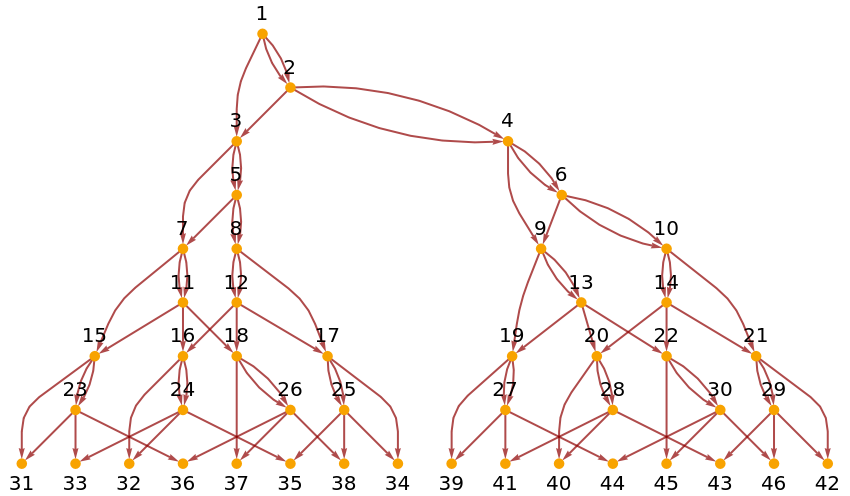Find the causal connection graph for events at step 3 (there are just two "subuniverses" in this case):

 In:=Out=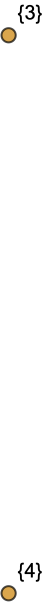Find the corresponding result for events at step 5:

 In:=Out=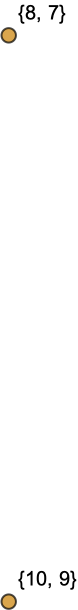Generate the causal connection graph for a “black hole”, where the future of a1 contains the future of a2, but not the other way around:

 In:=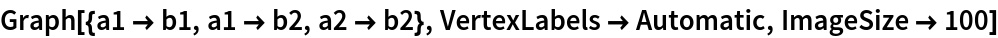Out=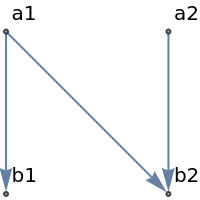In:=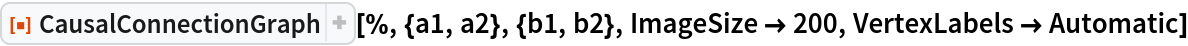Out=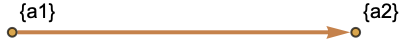### Scope (2)

Cases with undirected edges:

 In:=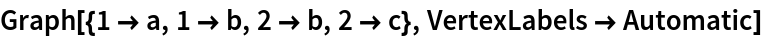Out=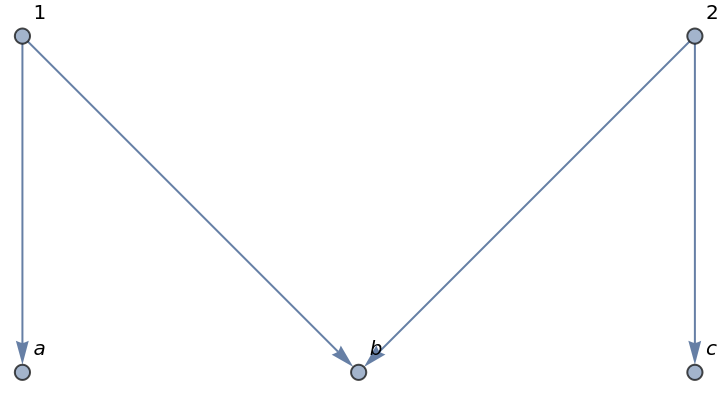In:=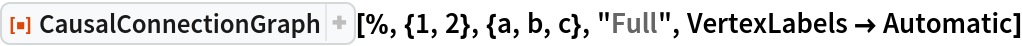Out=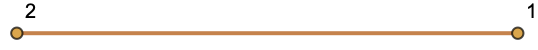Another example:

 In:=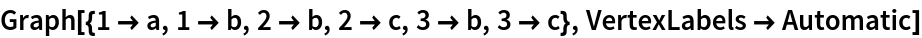Out=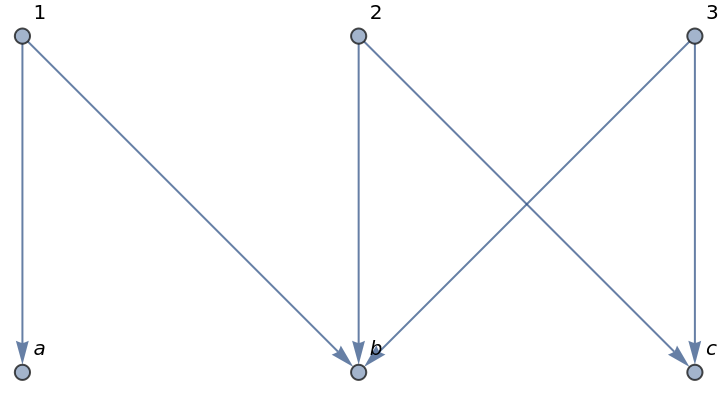In:=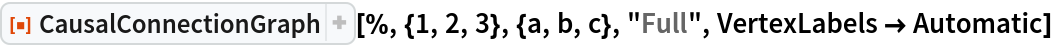Out=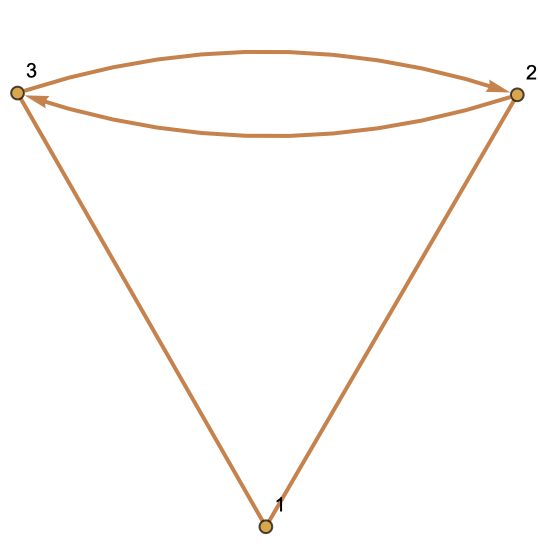## Version History

• 1.0.0 – 20 May 2020